# 【学习笔记】回归算法-线性回归

2019/03/28 10:54

[TOC]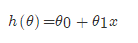，得出的值来判断一个瓜的好与不好的程度。通用公式为：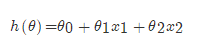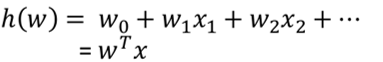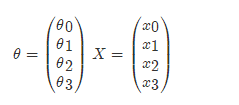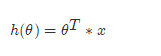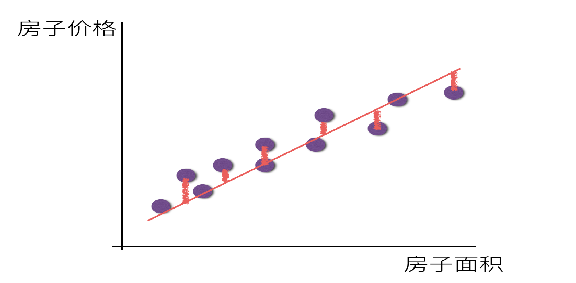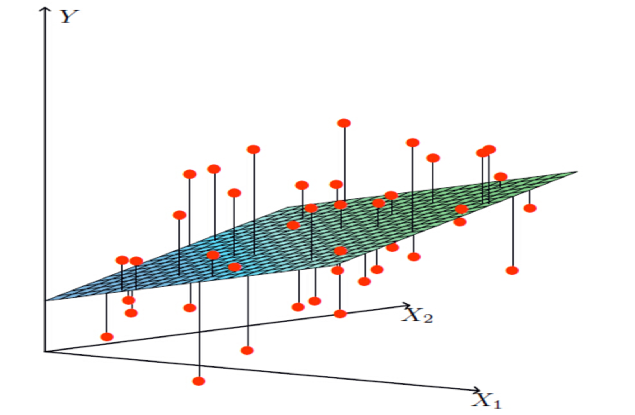## 损失函数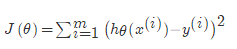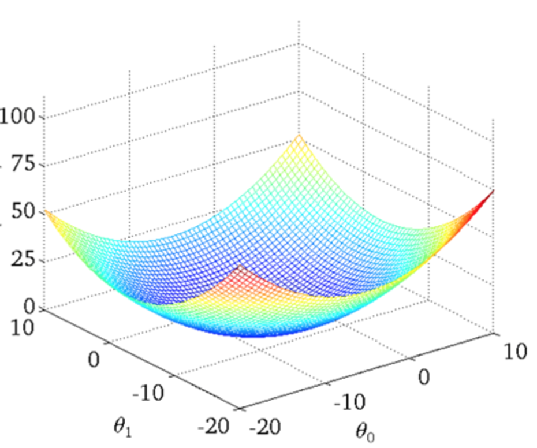## 正规方程## 梯度下降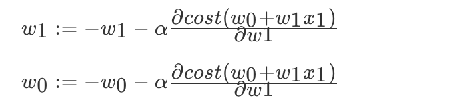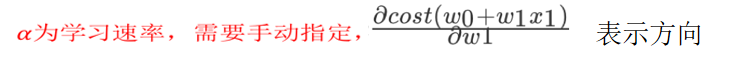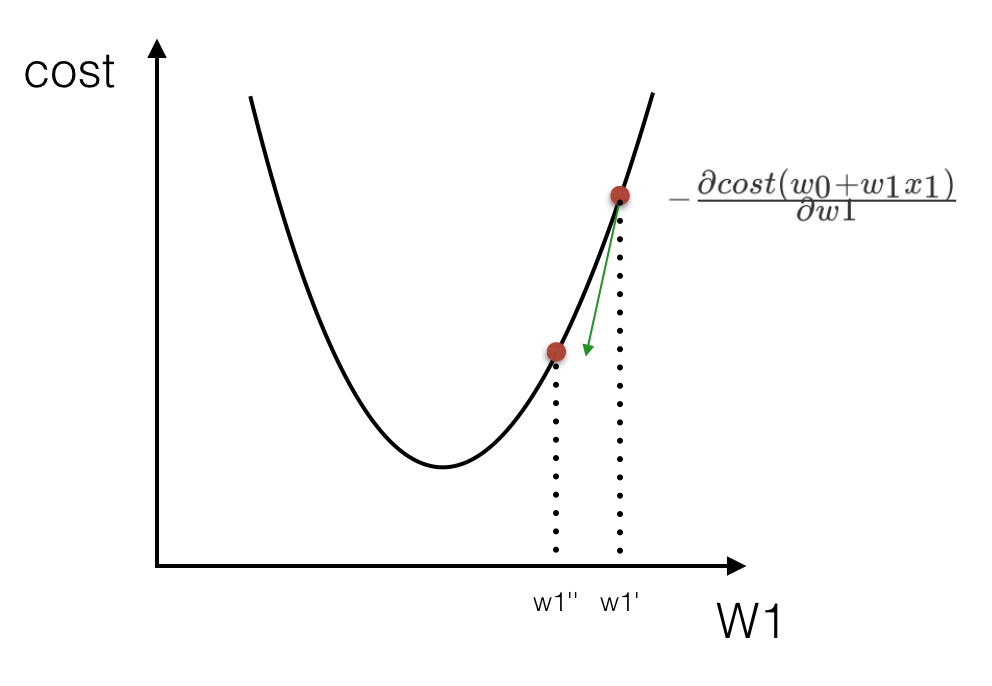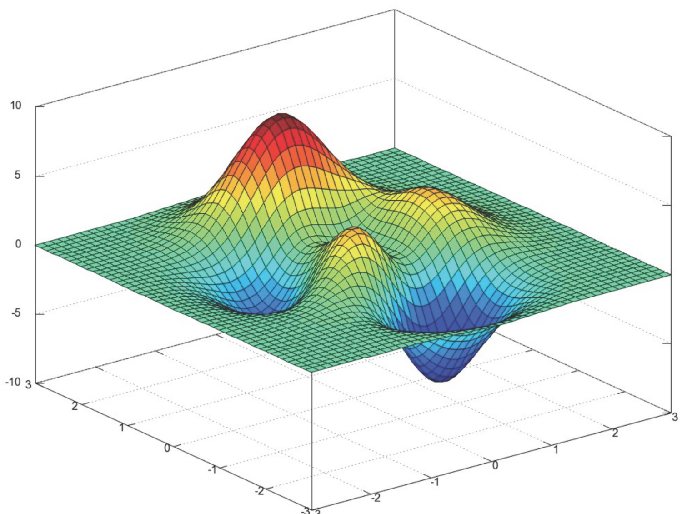## sklearn线性回归正规方程、梯度下降API

sklearn.linear_model.LinearRegression()

• 普通最小二乘线性回归
• coef_：回归系数

sklearn.linear_model.SGDRegressor( )

• 通过使用SGD最小化线性模型
• coef_：回归系数

from sklearn.datasets import load_boston
from sklearn.model_selection import train_test_split
from sklearn.preprocessing import StandardScaler
from sklearn.linear_model import LinearRegression, SGDRegressor

# 分隔数据集
x_train, x_test, y_train, y_test = train_test_split(lb.data, lb.target, test_size=0.25)

# 特征工程-标准化
std_x = StandardScaler()
x_train = std_x.fit_transform(x_train)
x_test = std_x.transform(x_test)
# 目标值
std_y = StandardScaler()
y_train = std_y.fit_transform(y_train.reshape(-1, 1))
y_test = std_y.transform(y_test.reshape(-1, 1))

# 正则方程求解预测结果
lr = LinearRegression()
lr.fit(x_train, y_train)
print(lr.coef_)
# 预测的价格
y_lr_predict = std_y.inverse_transform(lr.predict(x_test))
print("使用正规方程每个房子的预测价格：", y_lr_predict)

# 使用梯度下降进行预测
sgd = SGDRegressor()
sgd.fit(x_train, y_train)
print(lr.coef_)
y_sgd_predict = std_y.inverse_transform(sgd.predict(x_test))
print("使用梯度下降预测的房子价格：", y_sgd_predict)


## 回归性能评估

MSE的计算方法如下：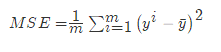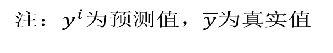### sklearn回归性能评估

mean_squared_error(y_true, y_pred)

• 均方误差回归损失
• y_true:真实值
• y_pred:预测值
• return:浮点数结果

from sklearn.metrics import mean_squared_error
...
print("正规方程的均方误差：", mean_squared_error(std_y.inverse_transform(y_test), y_lr_predict))
...

print("梯度下降的均方误差：", mean_squared_error(std_y.inverse_transform(y_test), y_sgd_predict))


正规方程的均方误差： 25.14886868419582



## 欠拟合与过拟合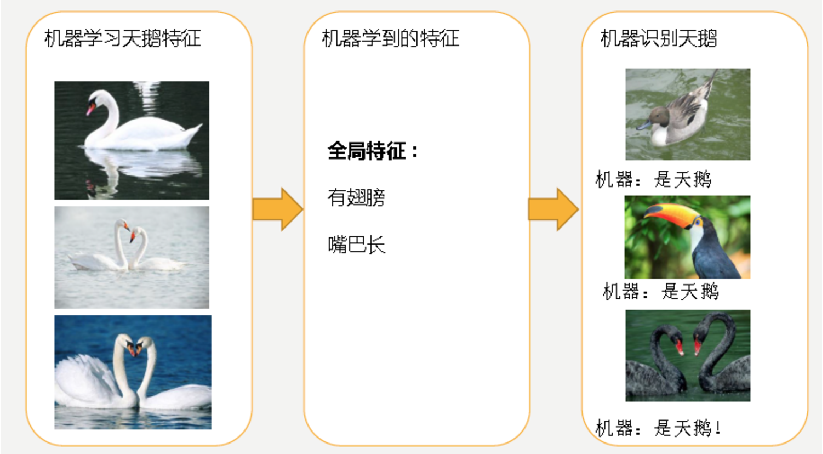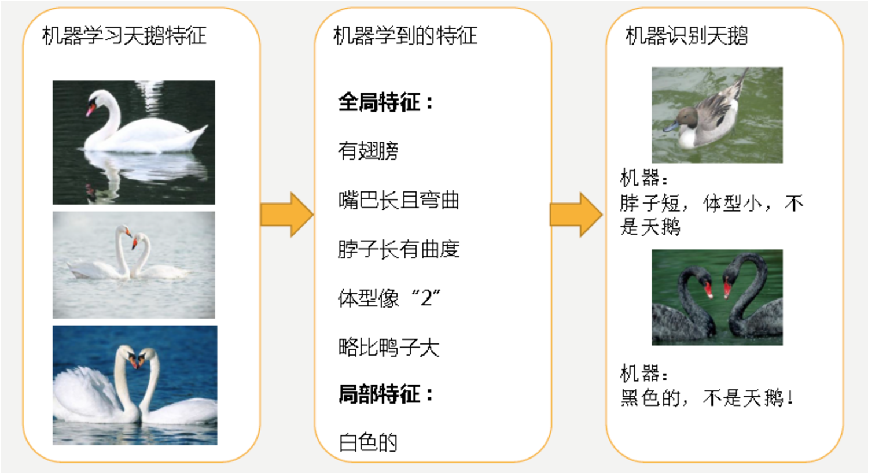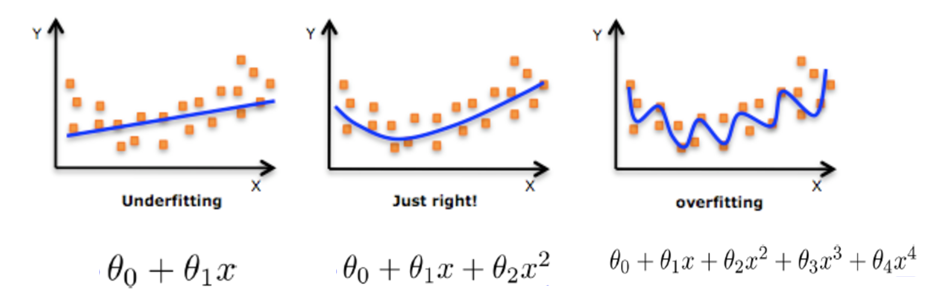### 解决过拟合的方法

#### 过拟合

• 进行特征选择，消除关联性大的特征(很难做)
• 交叉验证(让所有数据都有过训练)
• 正则化

## L2正则化

0
0 收藏

### 作者的其它热门文章0 评论
0 收藏
0